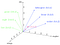# Cool Application of Word2Vec Model(in Python)

Firstly, let’s talk about what is a Word2Vec model. Word2Vec is one of the most popular techniques to learn word embeddings using a shallow neural network. It was developed by Tomas Mikolov in 2013 at Google. For the algorithm Odd One Out that we are going to implement soon, we will use the Google pre-trained model: ‘Googlenews-vectors-negative300.bin’, which can be downloaded from here. This model can be loaded using the gensim module, by the following code:

The model contains 300-dimensional vectors for 3 million words and phrases.

`samsung_vector = model["samsung"] #word vector of the word "samsung"apple_vector = model["apple"] #word vector of the word "apple"print(samsung_vector, apple_vector)((300,), (300,)) #printed result, both vectors are of 300 dimension.`

In this implementation, we will be using KeyedVectors(from gensim module) and cosine similarity function(provided by sklearn), import these two by the following code,

`from gensim.models import KeyedVectorsfrom sklearn.metrics.pairwise import cosine_similarity`

Now, let’s talk about the cool application of word2vec I’m talking about, its an algorithm named OddOneOut. What do I mean by OddOneOut? Let’s take an example so you can understand better. Assume we have a list of 5 words as [“apple”,” mango,” banana,” red,” papaya”]. If we have to tell which one of these five words is an odd one out, we can tell quickly tell it’s red because all the other words are names of fruits(all those words have the same context →fruits), that’s what we are going to implement. Our program will take input a list of words and then tell which word out of them is an odd one out.

The cosine similarity function will play a primary role in implementing this algorithm. What does cosine_similarity do? It computes similarity as the normalized dot product of X and Y. In simple words, we can use it to tell how much two terms are related to each other. Let us see by some examples,

`#similarity between the two words "samsung" and "apple" #here apple and samsung will be interpreted as mobile companies as context by the model.print(cosine_similarity([samsung_vector],[apple_vector]))array([[0.2928738]], dtype=float32) #Printed result`

As we can see, the similarity came out to be 0.29, which is close to zero. The more the cosine_similarity is close to zero more, the more the similarity is between the two words.Visual Image of word vectors of words with context as axes

Let’s discuss the algorithm of OddOneOut. What we are doing is passing a list of words to our program. So, what we will do is we will take the average of the word vectors of all the words, i.e., if word vectors of the words in the list are as v1,v2,v3……vn(n = no. of words in the list), the average vector can be found out by taking the mean of all the word vectors by np.mean([v1,v2,v3,…,vn],axis=0). Then we will set a variable mini and giving it a considerable high value, which will help in some comparisons we will see soon. Then we will commence a for loop and iterate over all the words in the list and will check the cosine similarity between each word with the avg vector we calculated. The word with the maximum value of similarity with the average vector will be our odd one out, as our average vector is made up of n-k words with the same context and k words (where k will be a small number) of a very different context from that of n-k words. By this, we are done with our implementation. You can follow the below code for implementing this algorithm.

I hope you liked this. Try implementing the algorithm on your own first. It’s a pretty cool algorithm, so I hope you had fun knowing about it. Keep Learning.

## Analytics Vidhya

### By Analytics Vidhya

Latest news from Analytics Vidhya on our Hackathons and some of our best articles! Take a look

## Analytics Vidhya

#### Analytics Vidhya is a community of Analytics and Data Science professionals. We are building the next-gen data science ecosystem https://www.analyticsvidhya.com